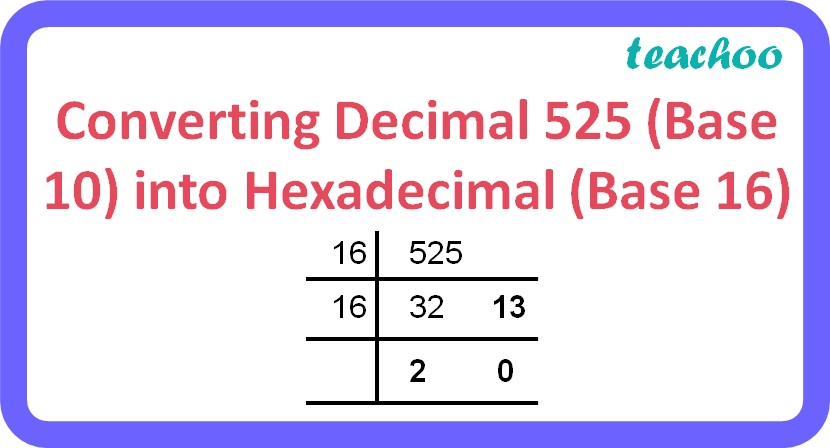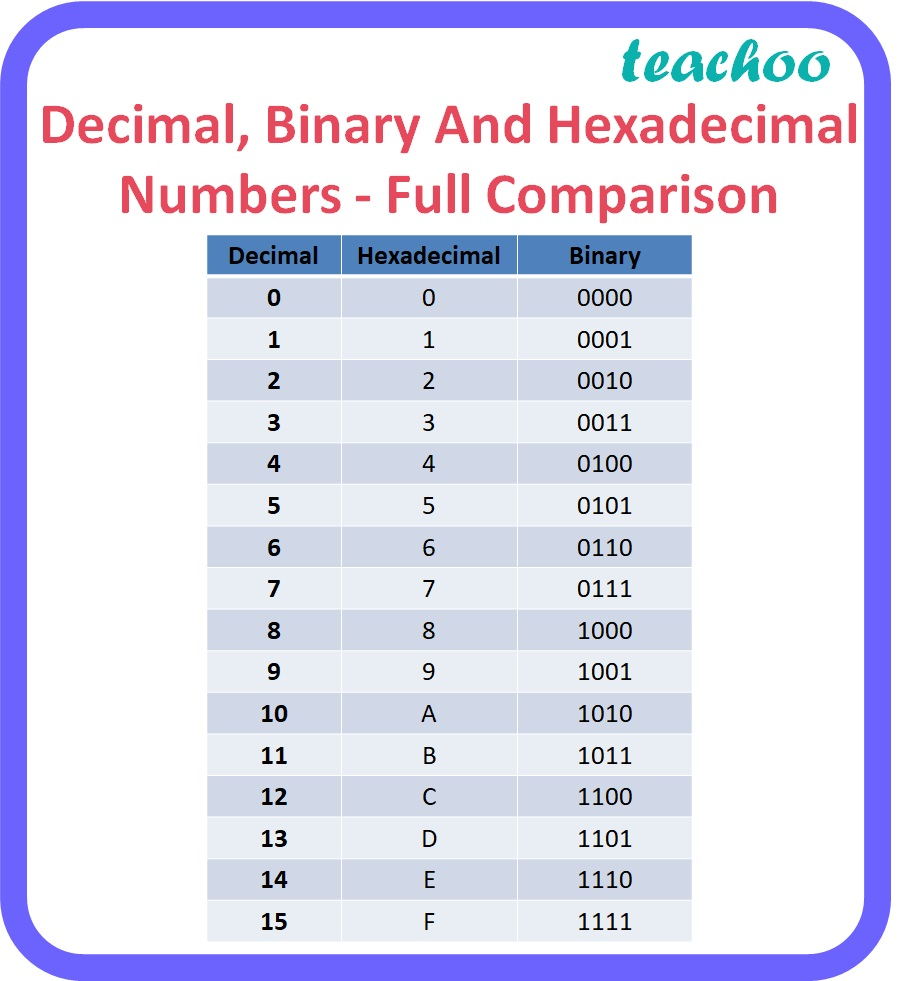3 Mark Questions

Computer Science - Class 11
Chapter 2 Class 11 - Encoding Schemes and Number System

## (b) 101101 2

• 525 10Therefore, 525 10 = 20D 16

Explanation:

• Repeatedly divide the decimal number by 16 until the quotient becomes less than 16 and record all the remainders. The remainders should be written bottom to upwards to get the hexadecimal equivalent of the decimal number.
• Here,
• 10 = A
• 11 = B
• 12 = C
• 13 = D
• 14 = E
• 15 = F

b. 101101 2

 00 10 1101
 2 D

Therefore, 101101 2 = 2D 16

Explanation:

• To obtain the Hexadecimal equivalent of a binary number, digits of binary number should be divided into groups of four digits starting from the rightmost digit for the integer part and starting from the leftmost digit for the fractional part.
• Convert each group of 4 digits to one hexadecimal digit as given in the table below.
• Note: 1,2 or 3 zeroes can be added before the leftmost digit or after the rightmost digit to make a complete group of 4 bits.Learn in your speed, with individual attention - Teachoo Maths 1-on-1 Class